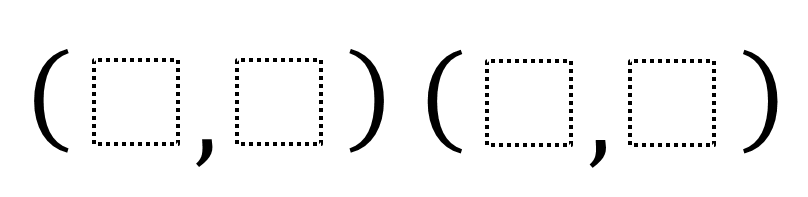# Perpendicular Lines and Slope

Directions: Fill in the boxes with the digits 1 through 9 so that the lines through each pair of points are perpendicular. Use each digit at most once.### Hint

What does it mean for lines to be perpendicular?
How can you compare pairs of points so that the lines going through the pairs of points are perpendicular?

Here are a couple sets of possible answers.
Line 1: (4, 9) and (3, 2)
Line 2: (1, 6) and (8, 5)

Line M: (2, 4) and (8, 6)
Line N: (3, 7) and (5, 1)

Source: Nanette Johnson

## Pythagorean Inequality

Directions: Use the whole numbers 1 through 6, at most one time each, to find …